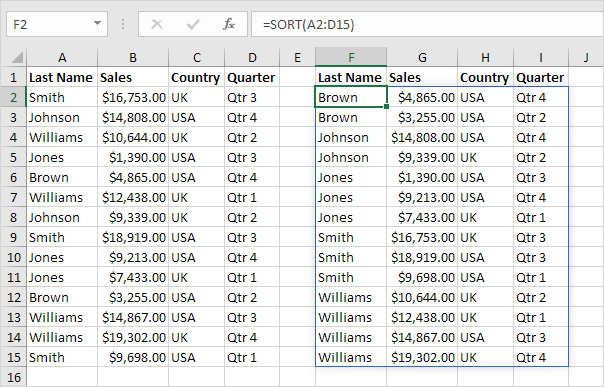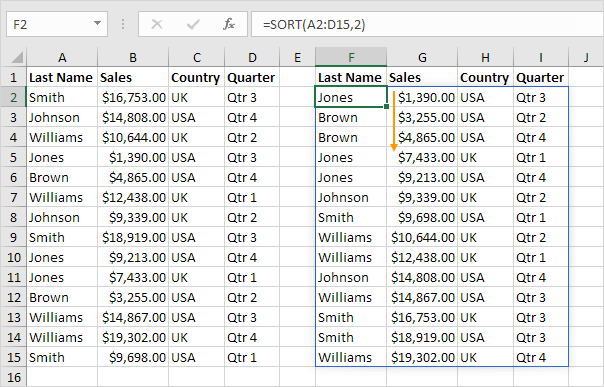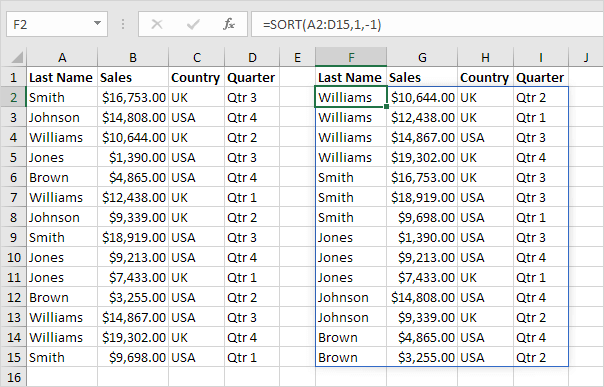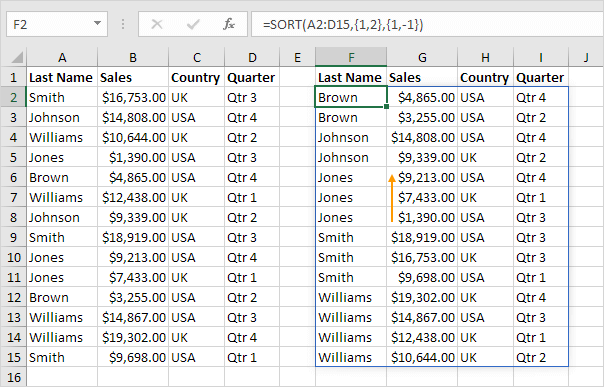# SORT function

Use the magic SORT function in Excel 365/2021 to sort your Excel data on one column or multiple columns. Let's give it a try.

1. The simple SORT function below has only 1 argument (A2:D15). By default, the SORT function sorts by the first column, in ascending order.Note: this dynamic array function, entered into cell F2, fills multiple cells. Wow! This behavior in Excel 365/2021 is called spilling.

2. Use the second argument of the SORT function to sort by a different column. The SORT function below sorts by the second column.Note: only edit the formula in cell F2. Excel will do the rest.

3. Use the third argument of the SORT function to sort in a different order. The SORT function below sorts by the first column, in descending order.Note: use 1 to sort in ascending order, use -1 to sort in descending order.

4. To sort on multiple columns, simply supply an array constant. For example, sort by Last Name first and Sales second.5. The advanced SORT function below sorts by Last Name first (in ascending order) and by Sales second (in descending order). Pretty cool, huh?6. Finally, use the SORTBY function in Excel to sort a range based on the values in a corresponding range.Note: use 1 to sort in ascending order, use -1 to sort in descending order.

Go to Next Chapter: Filter